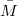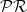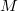﻿

### A general optimal inequality for warped product submanifolds in paracosymplectic manifolds

#### Abstract

The aim of this paper is to study the pseudo-Riemannian warped product submanifolds of a paracosymplectic manifold$\bar{M}$. We first, prove some fundamental lemmas and then derive some important results with parallel canonical structures on$\mathcal{P}\mathcal{R}$-semi-invariant submanifolds$M$ of$\bar{M}$. Finally, we describe the warped product submanifold$M$ of$\bar{M}$ by developing the general optimal inequality in terms of warping function and squared norm of second fundamental form. We also consider the totally geodesic, mixed geodesic and equality case of the inequality.

DOI Code: 10.1285/i15900932v37n2p45

Keywords: Paracontact manifold; Submanifold; Warped product

Full Text: PDF